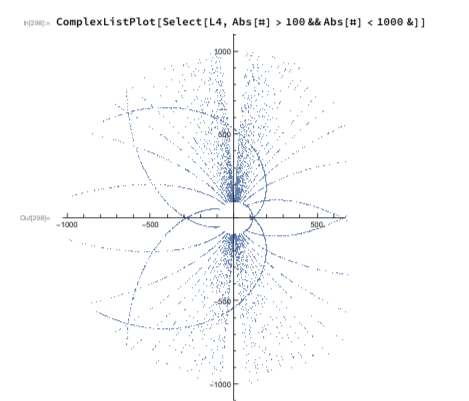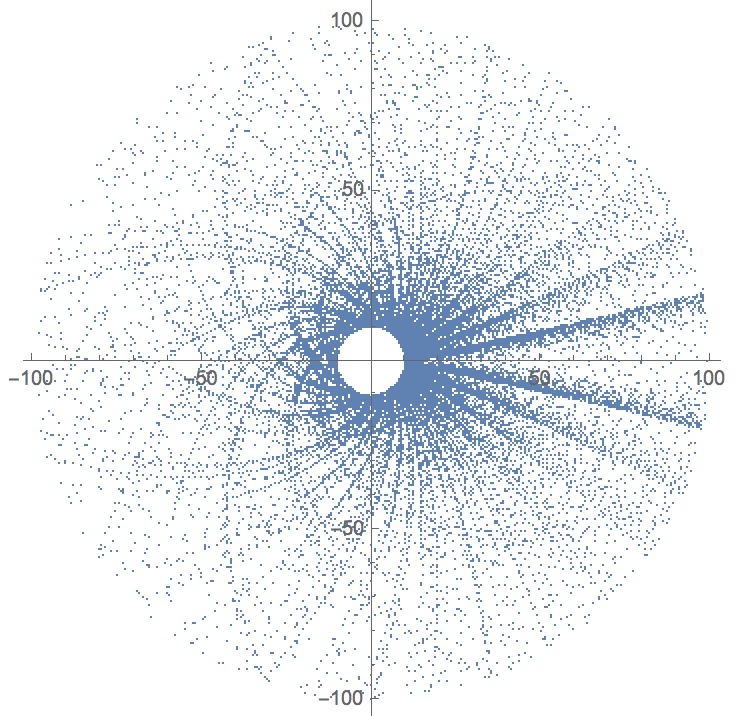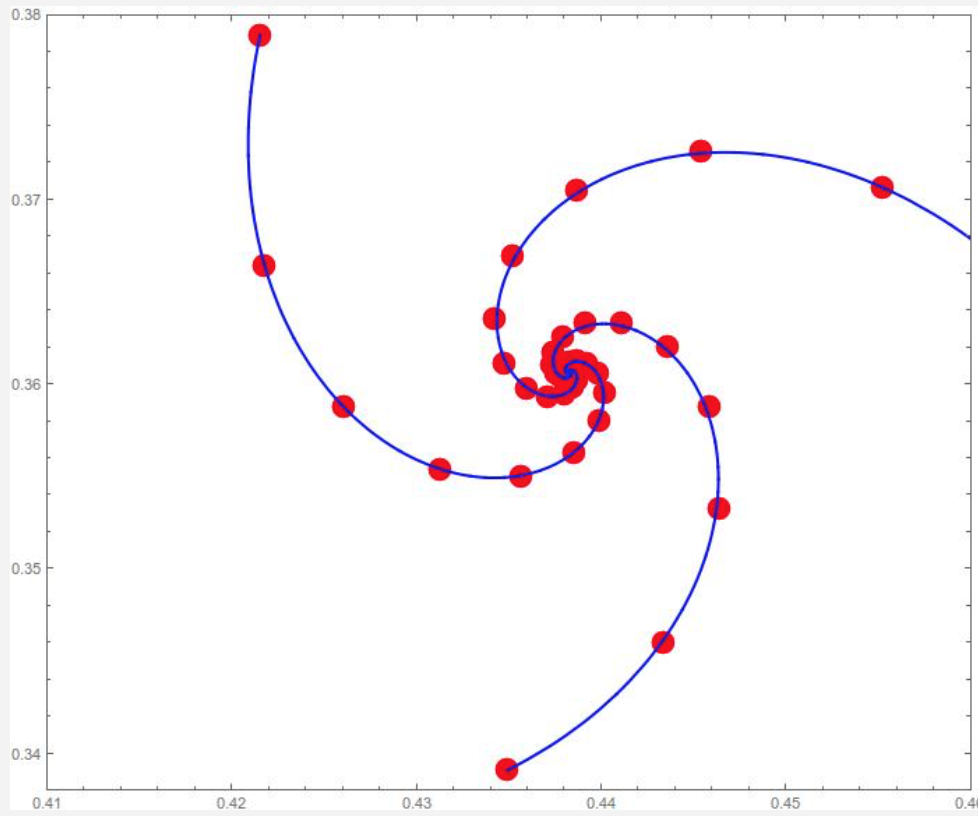# Exponential towers of $i$'s

It's well known that the expression $$i^i$$ takes on an infinite set of values if we understand $$w^z$$ to mean any number of the form $$\exp (z (\ln w + 2 \pi i n))$$ where $$\ln$$ is a branch of the natural logarithm function.

Since all values of $$i^i$$ are real, all values of $$i^{i^i}$$ (by which I mean $$i^{\left( i^i \right)}$$) are on the unit circle, and in fact they form a countable dense subset of the unit circle.

I can't figure out what's going on with $$i^{i^{i^i}}$$, though. I've posted a pdf version of Mathematica notebook at https://jamespropp.org/iiii.pdf containing images starting on page 2. Each image shows the points in the set of values of $$i^{i^{i^i}}$$ lying in an annulus whose inner and outer radii differ by a factor of 10. For instance, here's what we see in the annulus whose inner and outer radii are 100 and 1000 respectively.Can anyone see what's going on?

Also, what happens for taller exponential towers of $$i$$'s? Does the set of values of $$i^{i^{i^{{\ \kern3mu\raise1mu{.}\kern3mu\raise6mu{.}\kern3mu\raise12mu{.}\ }^i}}}$$ become dense in the complex plane once the tower is tall enough? The following picture makes me think that for a tower of height five, the set of values is everywhere dense.• Possibly relevant: Convergence of $i^{i^{i \ldots}}$ by Archibald James Macintyre [Proc. AMS 17 #1 (February 1966), p. 67]. – Dave L Renfro Apr 29 at 18:18
• When you have multiple (nested) exponentials do you mean to pick a common branch of log once for all before you apply any of them, or to chose a (possibly different) branch for each exponentiation? – Yaakov Baruch Apr 29 at 21:42
• I mean to choose a possibly different branch. – James Propp Apr 29 at 23:41
• For sets of complex numbers $A$ and $B$, let $A^B$ denote the set of all complex numbers of the form $\exp( z (\ln w + 2 \pi i n))$ wtih $w$ in $A$, $z$ in $B$, and $n$ an integer. Then I am asking about $A^{A^{A^A}}$ where $A = \{i\}$ (as well as taller towers of this form). – James Propp Apr 30 at 16:18
• on the wiki page List of mathematical constants the constant goes under the name infinite tetration $^\infty i$ of $i$ – Manfred Weis Apr 30 at 16:21

Let $$f : \mathcal{P}(\mathbb{C}) \rightarrow \mathcal{P}(\mathbb{C})$$ be the function on the powerset of the complex numbers defined by:

$$f(A) = \left\{ \exp\left( \frac{\pi i}{2}(4n + 1)z \right) : z \in A, n \in \mathbb{Z} \right\}$$

Then your question is asking about $$f^k(\{1\})$$ and its (topological) closure.

One thing to note is that, for any set $$A$$, we have $$\overline{f(A)} = \overline{f\left(\overline{A}\right)}$$, where the overline denotes closure. This follows from the fact that the expression $$\exp\left( \frac{\pi i}{2}(4n + 1)z \right)$$ is continuous as a function from $$\mathbb{C} \times \mathbb{Z}$$ to $$\mathbb{C}$$. So, if we want to study the closure of $$f^n(\{1\})$$, we are allowed to take the closure after each application of $$f$$.

The OP remarked that $$\overline{f^3(\{1\})}$$ is the unit circle. Then the set:

$$B :=\left\{ \frac{\pi i}{2}(4n + 1)z : z \in \overline{f^3(\{1\})}, n \in \mathbb{Z} \right\}$$

is precisely the union of a circle of radius $$\frac{\pi i}{2} k$$ for each odd integer $$k$$. Next, we need to take the image of this set $$B$$ under $$\exp$$. As the function $$\exp$$ is many-to-one, this is best understood by first reducing $$B$$ modulo $$2 \pi i$$ so that it lies in the strip with imaginary part $$[-\pi, \pi)$$.

Here's a picture of the first six circles in $$B \mod 2 \pi i$$:The intersection of $$B \mod 2 \pi i$$ with a line of fixed real part is a set of points which contains two limit points (the intersection with the light grey lines in the above plot). The closure of $$B \mod 2 \pi i$$ therefore contains all of these circles together with the two light grey lines (and nothing else).

$$\overline{f^4(\{1\})}$$ is therefore just the image of this set under $$\exp$$ (viewed as a bijective function from the strip to the punctured complex plane) together with the origin.

Now let's consider $$\overline{f^5(\{1\})}$$. Recall that the first step is to take the union of lots of homothetic copies of $$\overline{f^4(\{1\})}$$:

$$B' :=\left\{ \frac{\pi i}{2}(4n + 1)z : z \in \overline{f^4(\{1\})}, n \in \mathbb{Z} \right\}$$

If we pull this back through the inverse of the $$\exp$$ map (so we're working on the strip instead of the complex plane), this corresponds to taking the union of lots of translates of $$B \mod 2 \pi i$$. Specifically, we are interested in the set of points:

$$\log(B') :=\left\{ w + \log(i) + \log\left(\frac{\pi}{2}(4n + 1)\right) : w \in (B \mod 2 \pi i), n \in \mathbb{Z} \right\}$$

Now, the set $$\{ \log\left(\frac{\pi}{2}(4n + 1)\right) : n \in \mathbb{N} \}$$ has the very desirable property that the set is unbounded to the right and the gaps between the points become arbitrarily small. Also, the intersection of every horizontal line with the original set $$B \mod 2 \pi i$$ (the one in the picture above) is unbounded to the left. As such, it follows that their convolution is dense in every horizontal line, and therefore dense in the whole strip.

Consequently, $$\overline{f^5(\{1\})}$$ is the entire complex plane as you suspected.

The single-valued case of the exponential tower of $$i$$ was studied by Peter Lynch. The tower converges along three spirals to the fixed point $$Q=i^Q$$, given in terms of the Lambert W-function by $$Q=\frac{2i}{\pi}W\left(\frac{\pi}{2i}\right)=0.438\cdots+i\,0.361\cdots,$$ see image.• I'd guess this amazing constant is not linked to any other known constant? – Wolfgang May 5 at 17:34
• it's basically the Lambert W-function at $\pi/2i$, I don't know if it appears in other contexts. – Carlo Beenakker May 5 at 17:49
• I'd be very surprised if that evaluation of $W$ was exactly as you've written. Would not an $\approx$ be more appropriate? – David Roberts May 5 at 21:23
• you mean the numerical value of $W$ ? I only recorded the first few digits, more decimals are at oeis.org/A077589 and oeis.org/A077590 --- I added trailing $\cdots$ – Carlo Beenakker May 5 at 21:30